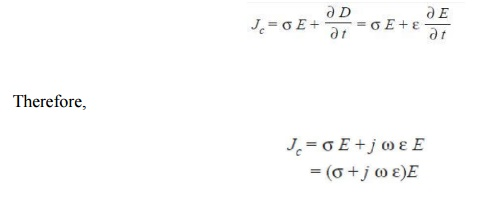Home | | High Voltage Engineering | Tan Delta Measurement

# Tan Delta Measurement

In general, in addition to conduction losses, ionizationand polarization losses also occur and, therefore, the dielectric constant ε = ε0 εr is no longer a real quantity rather it is a complex quantity.

TAN DELTA MEASUREMENT

In case of time varying electric fields, the current density Jc using Amperes law is given byIn general, in addition to conduction losses, ionizationand polarization losses also occur and, therefore, the dielectric constant ε = ε0 εr is no longer a real quantity rather it is a complex quantity. By definition, the dissipation factor tan δ is the ratio of real component of current Iω to the reactive component Ir (Fig.5.7). Here δ is the angle between the reactive component of current and the total current flowing through the dielectric at fundamental frequency. When δ is very small tan δ = δ when δ is expressed in radians and tan δ = sin δ = sin (90 – φ) = cos φ i.e., tan δ then equals the power factor of the dielectricmaterial.As mentioned earlier, the dielectric loss consists of three components corresponding to the three loss means for each of these an individual dissipation factor can be given such that mechanismεr* is called the complex relative permittivity or complex dielectric constant, ε′ and εr′ are called the permittivity and relative permittivity and ε″ and εr″ are called the loss factor and relative loss factor respectively.T he loss tangent the product of the angular frequency and ε″ is equivalent to the dielectric conductivity ζ″ i.e., ζ″ = ωε″. The dielectric conductivity takes into account all the three power dissipative processes including the one which is frequency dependent. Fig. 5.8 shows two equivalent circuits representing the electrical behavior of insulating materials under a.c. voltages, losses have been simulated by resistances

The loss tangentNormally the angle between V and the total current in a pure capacitor is 90°. Due to losses, thisangle is less than 90°. Therefore, δ is the angle by which the voltage and charging current fall short ofthe 90° displacement.

For the parallel circuit the dissipation factor is given byFor a fixed frequency, both the equivalents hold good and one can be obtained from the other. However, the frequency dependence is just the opposite in the two cases and this shows the limitedvalidity of these equivalent circuits.The information obtained from the measurement of tan δ and complex permittivity is an indicationof the quality of the insulating material.

If tan δ varies and changes abruptly with  the application of high voltage, it  shows inceptionof internal partial discharge.

The effect to frequency on the dielectric properties can be studied and the band of frequencies where dispersion occurs i.e., where that permittivity reduces with rise in frequency can be obtained.

Study Material, Lecturing Notes, Assignment, Reference, Wiki description explanation, brief detail
High Voltage Engineering : High Voltage Testing of Electrical Power Apparatus : Tan Delta Measurement |